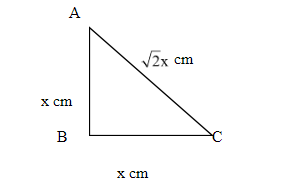Courses
Courses for Kids
Free study material
Free LIVE classes
MoreLIVE
Join Vedantu’s FREE Mastercalss

# If in a right angled isosceles triangle area of the triangle is 32 sq.cm. find all the sides of the isosceles triangle.Verified
360.9k+ views
Hint: In this question, the area of the triangle is given and also it is given that the triangle is a right angled isosceles triangle. So the method is that first of all we will find the area of the given triangle by assuming the sides as variable and then equate it to 32. On solving the equation we will get the sides.

Complete step-by-step solution -
In the question, it is given that area of the right angled isosceles triangle is 32 sq.cm.
And we have to find the sides of the triangle.
The diagram for question is shown below:In the above figure the triangle is a right angled isosceles triangle.
Let us assume that each of two equal sides = x cm.
We know that according to Pythagoras theorem in the $\vartriangle {\text{ABC}}$ , we can write:
${\text{A}}{{\text{B}}^2} + {\text{B}}{{\text{C}}^2} = {\text{A}}{{\text{C}}^2}$ .
Putting the values of AB and BC, we get:
${{\text{x}}^2} + {{\text{x}}^2} = {\text{A}}{{\text{C}}^2} \\ \Rightarrow {\text{AC = }}\sqrt {2{{\text{x}}^2}} = \sqrt 2 {\text{x}} \\$
We know that area of a right triangle is given as:
${\text{Area of }}\vartriangle {\text{ABC = }}\dfrac{1}{2} \times {\text{base}} \times {\text{height = }}\dfrac{1}{2} \times {\text{BC}} \times {\text{AB}}$ .

Putting the values of AB and BC in above equation, We get:
${\text{Area of }}\vartriangle {\text{ABC = }}\dfrac{1}{2} \times {\text{BC}} \times {\text{AB = }}\dfrac{1}{2} \times {\text{x}} \times {\text{x = }}\dfrac{{{{\text{x}}^2}}}{2}{\text{c}}{{\text{m}}^2}$
Now according to the question, we can write:
$\dfrac{{{{\text{x}}^2}}}{2} = 32 \\ \Rightarrow {{\text{x}}^2} = 64 \\ \Rightarrow {\text{x = }}\sqrt {64} = 8 \\$
Therefore, the length of the side AB=8cm.
And the length of the side BC= 8cm.
Length of side AC = $\sqrt 2 \times {\text{x = }}\sqrt 2 \times 8$ cm =11.31cm.

Note: In this type of question where the area of a triangle is given and the sides length is asked. First step is to draw the diagram of the question then assume the unknown side length. In this question the triangle is a right angled isosceles triangle. So you should know the area of the isosceles right triangle.
Last updated date: 28th Sep 2023
Total views: 360.9k
Views today: 11.60k